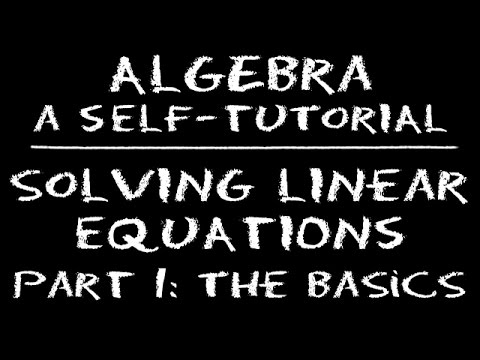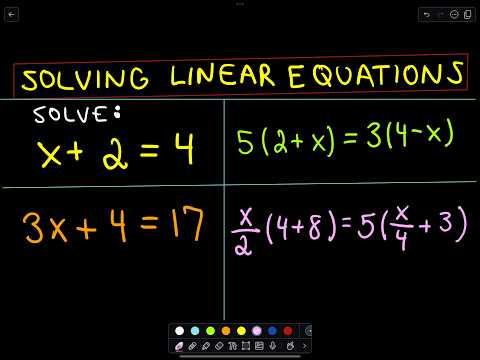## Linear Equation

Subject: Compulsory Maths

Find Your Query

#### Overview

A linear equation is an algebraic equation in which each term is either a constant or the product of a constant and (the first power of) a single variable.

### Solving Simultaneous Linear EquationsThere are two variables x and y in the above pair of equations. The given pair of linear equations represents a pair of straight lines while plotting on the graph. The method of obtaining the value of the two variables (order Pairs) which satisfy both the equations is known as ' solution of equations '. If a pair of values of two variables involved in a pair of equations satisfies both the equations, those equations are called linear simultaneous equation. There are many methods of solving such pair of linear equations.

To solve a system of linear equations by elimination method:

1. Make equal coefficients of either x or y, multiply one or both equations by a constant.
2. Add or subtract the respective sides of the equations to eliminate either of the variables.
3. Solve for the variables and substitute the value found into either of the original equations to find the value of the remaining variable.

To solve a system of linear equation by substitution method:

1. Take one of the two equations and express y in terms of x or x in terms of y.

2. Now, substitute the value of y (or x) in another equation and solve it to get the value of x (or y).

3. Substitute the value of x (or y) so obtained in any one equation and solve it to get the value of y (or x).

To obtain the solution of a pair of a equations a1x + b1y and a2x + b2y = c2by graphical method:

1. Construct tables of ordered pairs giving the different independent value of x in order to get corresponding value of y.

2. Graph each ordered pairs of the equation and get a pair of straight lines.

3. Determine the point of intersection. Ordered pair representing the point of the intersection is the solution of both the equation.

4. The graph of the simultaneous equation is always a straight line. Thus, such equation is called linear equations.

##### Things to remember
• Simultaneous equation consists of two variables'x' and 'y'
• Value of x and y are ordered pair that should satisfy both equations
• There are three major methods to solve a linear simultaneous equation. They are:- Substitution method, Elimination method and Graphical Method.

• It includes every relationship which established among the people.
• There can be more than one community in a society. Community smaller than society.
• It is a network of social relationships which cannot see or touched.
• common interests and common objectives are not necessary for society.
##### Videos for Linear Equation##### Algebra: Linear equations 1 | Linear equations | Algebra I | Khan Academy##### Algebra: Solving Linear Equations - Part 1: The Basics##### Questions and Answers

Here,

given equation,x = 3y

x-3y=0.........(i) and

4x+y=26........(ii)

Multiplying equation (i) by 4 and subtracting equation (ii) from (i),we get,

4x-12y=0

4x+y=26

$\frac{-\;-\;-\;}{-13 y =-26}$

∴y=$\frac{-26}{-13}$=2

Here,putting y=2 in equation (i),we get

x - 3 × 2 =0

∴x=6

Hence, x=6 and y=2 Ans

To verify:Substituting the value of x and y in equation (i) and (ii)

(i) 6-3×2 =6-6=0(True)

(ii) 4× 6 + 2 = 24 +2 =26(True)

Here,

Given equation

x+y=-5.........(i) and

-3x+2y=0

3x-2y=0..........(ii)

Subtracting equation (ii) from equation obtained by multipling equation(i) by 3.

3x+3y=-15

3x-2y=0

$\frac{-\;+\;-\;}{-5 y =-15}$

∴y=$\frac{-15}{-5}$=-3

Here,substituing the value of y=-3in equation (i),we get

x - 3 =-5

∴ x=-5+3=-2

Hence, x=-2 and y=-3Ans

To verify:Substituting the value of x and y in equation (i) and (ii)

(i) -2-3 =-5(True)

(ii) 3× (-2) -2×(-3)= -6+6=0(True)

Here,

Given equation

2x+y=11.........(i) and

x+3y=18..........(ii)

Subtracting equation (i) from equation obtained by multipling equation(ii) by 2,we grt

2x+6y=36

2x+y=11

$\frac{-\;+\;-\;}{5 y =25}$

∴y=$\frac{25}{5}$=5

Now, putting the value of y=5in equation (i),we get

2x + 5 =11

or, 2x=11-5

∴x=$\frac{6}{2}$=3

∴x=3 and y=5 Ans.

To verify:Substituting the value of x and y in equation (i) and (ii)

(i) 2 × 3 + 5=6+5=11(True)

(ii) 3+3 ×5=3+15=18(True)

Here,

Given equation

x+y=6.........(i)

and 2x-y=3..........(ii)

Adding equation (i) and (ii) ,we get

x+y=6

2x-y=3

$\frac{\;\;\;}{3 x =9}$

∴x=$\frac{9}{3}$=3

Here, Substitute the value of x in equation (i),

3 + y =6

∴ y=6-3 = 3

Hence, x=3 and y=3 Ans

To verify:Substituting the value of x and y in equation (i) and (ii), we get

(i) 3 + 3=6(True)

(ii) 2 × 3 -3= 6-3 =3(True)

Here,

Given equation

x+2y=9.........(i)

and x=2y+1

or, x-2y=1..........(ii)

Adding equation (i) and (ii) ,we get

x+2y=9

x-2y=1

$\frac{\;\;\;}{2 x =10}$

∴x=$\frac{10}{2}$=5

Here, putting x=5in equation (ii),we get

5 - 2y =1

or, 5-1=2y

or,4=2y

∴y=$\frac{4}{2}$=2

∴x=5 and y=2.Ans.

To verify:Substituting the value of x and y in equation (i) and (ii), we get

(i) 5 + 2 × 2=5+4=9(True)

(ii) 5-2×2=5-4=1(True)

Here,

Given equations,

2x+y=3...............(i)

and 2x-y=1.............(ii)

from above equation (i),

2x+y=3

or, y=3-2x

Now, construct a table by assigning the different values of x in order to get the value for y .

 x 1 0 -1 2 y 1 3 5 -1figure

Again, from equation (ii)

2x-y=-1

or, 2x-1=y

Now, construct a table by assigning the different values of x in order to find the values of y.

 x 0 1 2 -2 y -1 1 3 -5

In above graph, the st.line obtain from equation (i) and (ii) intersect at the point(1,1). Hence, the required solution of given equations are x=1 and y=1.

To Verify:

2x+y=3......(i)

or, 2 × 1 =1=3

or, 2 + 1 =3

or,3=3(True)

2x-y=1..............(ii)

or, 2×-1=1

or, 2-=1

or, 1 =1(True)

Here,

Given equations,

x+2y=8...............(i)

and 2x-y=-2.............(ii)

from equation(i),

x+2y=8

or, x=8-2y

Now, construct a table by assigning the different values for y in order to get the value of x.

 x 6 4 2 0 y 1 2 3 4figure

Again, from equation (ii)

2x-y=-2

or, 2x+2=y

Now, construct a table by assigning the different values of x in order to find the values of y.

 x 1 -1 -2 0 y 4 0 -2 2

In above graph, the st.line obtain from equation (i) and (ii) intersect at the point(0.8,3.6). Hence, x =0.8 and y =3.6 are the required solution of given equations.

Verification:

x+2y=8......(i)

or, 0.8 + 2× 3.6 =8

or, 0.8+ 7.2 = 8

or, 8=8(true)

2x-y=-2..............(ii)

or, 2× 0.8 -3.6=-2

or, 1.6-3.6=-2

or, -2 =-2(True)

Here,

Given equations,

x-2y=-1...............(i)

and 2x-y=-4.............(ii)

From above equation (i),

x-2y=-1

or, x=2y-1

Now, construct a table by assigning the different values of y in order to calculate the value of x.

 x -1 1 3 -3 y 0 1 2 -1

Again, from equation (ii)

2x-y=-4

or, 2x+4=y

Now, construct a table by assigning the different values of x in order to find the values of y.

 x 1 0 -1 -2 y 6 4 2 0figure

I

n above graph, the st.line obtain from equation (i) and (ii) intersect at the point($\frac{-7}{-2}$). Hence, x =$\frac{-7}{3}$ and y =$\frac{-2}{3}$ are the required solution of given equations.

To Verify:

x-2y=-1......(i)

or, $\frac{-7}{3}$-2($\frac{-2}{3}$)=-1

or,$\frac{-7}{3}$+$\frac{4}{3}$=-1

or, $\frac{-7+4}{3}$=-1

or, $\frac{-3}{3}$=-1

or,-1=-1(True)

2x-y=4...........(ii)

or, 2×

Here,

Given equation

-x+3y=8.........(i)

and x+2y=-13..........(ii)

Adding equation (i) and (ii) ,we get

-x+3y=8

x+2y=-13

$\frac{\;\;\;}{5 y =-5}$

∴y=$\frac{-5}{5}$=-1

Put y=-1 in equation (i).

-x+3×(-1)=8

or,-x=8+3

or,-x=11

∴x=-11

Hence, x=-11 and y=-1 Ans

To verify:Substituting the value of x and y in equation (i) and (ii), we get

(i) -(-11)+3×(-1)=11-3=8(True)

(ii) -11+2×(-1)=-11-2=-13(True)

Here,

Given equation

8x + 5y =2 .........(i)

and 7x +4y =-1..........(ii)

By multiplying equation (i)by 4 and (ii) by 5 ,we get

-32x+20y=8

35x+20y=5

$\frac{\;-\;-\;-}{-3 x=3}$

∴x=$\frac{-3}{3}$=-1

Here, Put x=-1 in equation (ii),then

7×(-1)+4y=1

or,-7+4y=1

or,y=$\frac{1+7}{4}$

∴y=2

∴x=-1 and y=2 Ans

Verification:Substituting the value of x and y in equation (i) and (ii), we get

(i) 8×(-1)+5×2=-8+10=2(True)

(ii) 7×(-1)+4×2=-7+8=1(True)

Here,

Given equation

2x - 3y =1 .........(i)

and x =y +2

or, x-y=2..........(ii)

Subtracting from (i) by multipllying the equation (ii) by 2 ,we get

-2x+3y=1

2x-2y=4

$\frac{\;-\;+\;-}{-y=-3}$

∴y=3

Here, Put y=3 in equation (ii)

x-3=2

∴x=2+3=5

Hence,x=5 and y=3 Ans.

To verify: Substituting the value of x and y in equation (i) and (ii), we get

(i) 2×5-3 ×3=10-9=1(True)

(ii) 5-2=2(True)

Here,

Given equation

2x - 3y =1 .........(i)

and x =y +2

or, x-y=2..........(ii)

Subtracting from (i) by multipllying the equation (ii) by 2 ,we get

-2x+3y=1

2x-2y=4

$\frac{\;-\;+\;-}{-y=-3}$

∴y=3

Here, Put y=3 in equation (ii)

x-3=2

∴x=2+3=5

Hence,x=5 and y=3 Ans.

To verify: Substituting the value of x and y in equation (i) and (ii), we get

(i) 2×5-3 ×3=10-9=1(True)

(ii) 5-2=2(True)

Here,

Given equation

y=2x+3.........(i)

or, -2x+y=3

3x+2y=20.......(ii)

Multiplying equation (i) by 2 subtracting from equation (ii) ,we get

-3x+2y=20

or, -4x+2y=6

$\frac{\;+\;-\;-}{7x=14}$

∴x=$\frac{14}{7}$=2

Here, Putting x=2 in equation (ii) we get

-2×2+y=3

or, -4+y=3

∴x=2and y=7 Ans.

Verification: Substituting the value of x and y in equation (i) and (ii), we get

(i) -2×2+7=-4+7=3(True)

(ii) 3×2+2×7=6+14=20(True)

##### Quiz

© 2019-20 Kullabs. All Rights Reserved.